Article

Audio Encryption Algorithm Based on Chen Memristor Chaotic System

1
School of Mathematics and Computing Science, Guilin University of Electronic Technology, Guilin 541004, China
2
School of Data and Statistical Sciences, Xinjiang University of Finance and Economics, Urumqi 830012, China
3
School of Information and Software Engineering, University of Electronic Science and Technology of China, Chengdu 610054, China
4
Guangxi Colleges and Universities Key Laboratory of Data Analysis and Computation, Guilin University of Electronic Technology, Guilin 541004, China
*
Author to whom correspondence should be addressed.
Symmetry 2022, 14(1), 17; https://doi.org/10.3390/sym14010017
Received: 19 October 2021 / Revised: 14 December 2021 / Accepted: 16 December 2021 / Published: 23 December 2021
(This article belongs to the Special Issue Discrete and Continuous Memristive Nonlinear Systems and Symmetry)

Abstract

The data space for audio signals is large, the correlation is strong, and the traditional encryption algorithm cannot meet the needs of efficiency and safety. To solve this problem, an audio encryption algorithm based on Chen memristor chaotic system is proposed. The core idea of the algorithm is to encrypt the audio signal into the color image information. Most of the traditional audio encryption algorithms are transmitted in the form of noise, which makes it easy to attract the attention of attackers. In this paper, a special encryption method is used to obtain higher security. Firstly, the Fast Walsh–Hadamar Transform (FWHT) is used to compress and denoise the signal. Different from the Fast Fourier Transform (FFT) and the Discrete Cosine Transform (DCT), FWHT has good energy compression characteristics. In addition, compared with that of the triangular basis function of the Fast Fourier Transform, the rectangular basis function of the FWHT can be more effectively implemented in the digital circuit to transform the reconstructed dual-channel audio signal into the R and B layers of the digital image matrix, respectively. Furthermore, a new Chen memristor chaotic system solves the periodic window problems, such as the limited chaos range and nonuniform distribution. It can generate a mask block with high complexity and fill it into the G layer of the color image matrix to obtain a color audio image. In the next place, combining plaintext information with color audio images, interactive channel shuffling can not only weaken the correlation between adjacent samples, but also effectively resist selective plaintext attacks. Finally, the cryptographic block is used for overlapping diffusion encryption to fill the silence period of the speech signal, so as to obtain the ciphertext audio. Experimental results and comparative analysis show that the algorithm is suitable for different types of audio signals, and can resist many common cryptographic analysis attacks. Compared with that of similar audio encryption algorithms, the security index of the algorithm is better, and the efficiency of the algorithm is greatly improved.

1. Introduction

With the rapid development of the internet, wireless voice communication technology was widely applied in real-life, and in the process of data transmission there is the risk of information leakage; therefore, audio information encryption research is of great significance. Many traditional encryption algorithms are widely used in audio encryption and achieved good encryption results, such as Advanced Encryption Standard (AES) [1,2], Data Encryption Standard (DES) [3,4] and S-box algorithm [5,6], etc., Although these algorithms have perfect encryption technology, some algorithms still have some defects and cannot fully ensure the security of encryption. The main disadvantage of AES is that it uses a static S-box in the whole algorithm, which damages the security of AES and may be subject to different algebraic attacks. Therefore, to overcome this shortcoming, Amandeep S.  and others proposed a dynamic S-box AES encryption algorithm. Because the chaotic system has the characteristics of ergodicity, initial value sensitivity, and strong pseudo-random sequence, many scholars proposed to encrypt plaintext information by combining the AES algorithm with the chaotic system, and the results were satisfactory. Alireza A.  et al. Proposed to encrypt the image by combining the chaotic sequence with the improved AES algorithm. The experimental results show that this encryption method reduces the time complexity of the algorithm, increases the keyspace of the algorithm, and significantly improves security. The chaotic system has made an important contribution to the encryption scheme. Therefore, in recent years, chaotic systems were widely used in encryption algorithms [9,10,11]. Abdelfatah R.I.  proposed a multichaotic mapping audio encryption scheme, and the novel features of this algorithm are as follows: adaptive scrambling and cryptographic feedback are used to realize global scrambling and deep diffusion, respectively. At the same time, four different audio encryption technologies are combined in the same scheme to make it more secure. Shah D. et al.  proposed that Mobius transform was used as the source to generate a strong S-box substitution network and Henon chaotic map to perform pixel-level displacement. However, the Henon chaotic map has a low dimension, and its keyspace is limited. To solve this problem, Zhao H. et al.  proposed an adaptive symmetric Henon chaotic map, which has the characteristics of wide parameter range and high complexity. Therefore, it has a larger keyspace and is more suitable for encryption algorithms. Farsana F.J. et al.  introduced a modified discrete Henon map, which can weaken the correlation between adjacent samples. At the same time, an improved super-Lorenz chaotic system was proposed to iterate and fill the silent period in speech conversation, and a dynamic key flow mechanism was designed to enhance the correlation between Ming and ciphertext. Some audio encryption schemes meet the security requirements of the design by enhancing the complexity of the algorithm but increasing the difficulty of data processing and the time of audio encryption, such as Naskar P.K. et al.  proposed an audio encryption algorithm based on DNA Encoding and Channel Shuffling, which overcomes the inefficient algorithm of multiple rounds. However, due to the adoption of an encryption scheme based on traditional mathematical methods, the timeliness of the encryption algorithm is reduced. Of course, in the existing research, there is no lack of many encryption algorithms that were decoded. Saeed N. et al.  found the loopholes through simulation, obtained the key of the algorithm, and decoded an image encryption algorithm related to chaotic mapping. This is a good example.
A memristor is a kind of nonlinear element with memory function and nanoscale size. It can be used as a nonlinear part of a chaotic system and has rich dynamic behaviors, which significantly improves the randomness and complexity of chaotic system signals . Because the memristor is a controllable nonlinear device, in some systems, the chaotic attractors of the even and singular vortices can be obtained only by changing the parameters of the memristor. Therefore, the memristor chaotic system can provide a complex, variable, and reliable pseudo-random cipher generator for the encryption algorithm and can effectively avoid the degradation of the chaotic system dynamics.
Bao B.C.  designed and studied a chaotic circuit based on memristor. The active double-ended memristor circuit is used to replace Chua’s diode. The circuit is directly extended from Chua’s oscillator to obtain a new chaotic system. The results show that the introduction of memristor makes the dynamic behavior of the system more complex. Ma Xujiong  proposed a chaotic circuit with memristor, mode resistance capacitor, and linear inductor established the dimensionless mathematical model of the circuit and found that 19 different types of chaotic attractors will be generated in the circuit. Compared with that of the traditional chaotic attractors, the circuit has rich dynamic characteristics and good application prospects in the field of secure communication. Chen J.J.  designed an image encryption algorithm of a new hyperchaotic system based on memristor, which can produce complex chaotic attractors. The experimental results show that the circuit simulation and numerical simulation results of the system always proved the feasibility, effectiveness, and ability to produce chaotic behavior of the system. Combined with the proposed image encryption scheme, the security analysis shows that the scheme is not easy to crack and can resist all kinds of attacks. Peng G.  proposed a new modal memristor chaotic system, realized its circuit simulation, and applied it to image encryption. The application scope of the memristor is far beyond these. For example, Kamal F.M.  designed a new fractional nonautonomous chaotic circuit model by introducing a fractional element memristor circuit; Akgul A.  proposed a new fractional-order chaotic circuit with memristor and linear inductor. To show the application advantages of the proposed chaotic system, it also realized the synchronization of fractional-order chaotic system and applied it to secure communication system for the first time. In addition, in their latest research, Yan D.  also proposed a chaotic attractor based on the combination of fractal transformation and memristor chaotic system. The main advantage of the system is that multiple rolling attractors and extended chaotic attractors can be generated only by modifying system parameters, and various circular chaotic attractors can be generated in combination with classical Julia fractal. Its attractor has complex dynamic behavior , which is very suitable for all kinds of secure communication fields.
In recent years, with the in-depth study of memristor chaotic system, it is more and more widely used in encryption algorithms. To improve the security and efficiency of digital speech encryption algorithm, an audio encryption algorithm based on Chen memristor chaotic system is proposed in this paper. To further improve the security of the traditional scrambling and diffusion encryption framework, a special audio encryption method is proposed. The Fast Walsh–Hadamard Transform is used for adaptive compression, denoising, and reconstruction of the audio signal. The high complexity chaotic mask block is used as the filling layer, and the dual-channel audio signal is cleverly transformed into a color audio image. To make the encryption algorithm highly correlated with plaintext audio, the audio’s stored byte information is taken as the parameter of interactive channel shuffling operation, and the cipher block is used for overlapping diffusion encryption to obtain ciphertext audio. Ciphertext audio is transmitted in the form of a noise image so that the algorithm has two protective barriers, which can effectively reduce the risk of ciphertext audio information being intercepted and cracked in the transmission process. In addition, compared with that of Chen chaotic system, the Chen memristor chaotic system has a larger bifurcation interval in the range of parameter c, which means that it has a larger keyspace and can resist violent attacks. The encryption scheme not only solves the security problems of simple scrambling and diffusion operation but also improves the efficiency of the encryption algorithm.
Our contributions are as follows:
(1) The Chen memristor chaotic system has a larger chaotic parameter interval and can produce pseudo-random sequences with high complexity, which is more difficult to predict than general chaotic signals.
(2) A method of skillfully transforming the audio signals into image information is proposed.
(3) We propose an audio encryption algorithm based on interactive channel shuffling and overlapping diffusion. Experimental simulation shows that the algorithm has high security.
The rest of this paper is organized as follows: in Section 2, a new memristor chaotic system model is proposed and the correlation dynamics are analyzed; in Section 3, an encryption algorithm for compressing and denoising audio signals and transforming them into image information is proposed. In the algorithm, interactive channel shuffling and overlapping diffusion are also designed; in Section 4, the experimental simulation and security analysis of the encryption algorithm is carried out; and Section 5 summarizes and discusses the full text.

2. Model and Dynamic Analysis of a Novel Memristor Chaotic System

2.1. Chen Chaotic System Model Based on Magnetically Controlled Titania Memristor

There is an insulating layer of TiO$2$ film and a conductive layer of TiO$2 − x$ film between the two platinum electrodes. The pure TiO$2$ layer has high resistivity, while TiO$2 − x$ material is conductive due to oxygen vacancy. When the current flows through the device in one direction, the boundary between the two materials moves, increasing the percentage of conductive layer TiO$2 − x$. At this time, the resistance of the memristor decreases. When the current direction is opposite, the boundary will move in the opposite direction, the percentage of TiO$2$ (insulating layer) increases, and the resistance of the memristor increases. When the current stops, the boundary stops moving, and the boundary between the two layers of the memristor freezes and maintains the last resistance value. In other words, the memristor “remembers” the current flowing through it. The physical model of the memristor  is shown in Figure 1.
The known dynamic equation of the Chen chaotic system  is
$x ˙ = a ( y − x ) , y ˙ = ( c − a ) x − x z + c y , z ˙ = x y − b z .$
where a, b, c are the system parameter and its values are $a = 35$, $b = 3$, $c = 28$, respectively, the system is in a chaotic state.
Memristor is a nonlinear electronic component and has great potential in chaotic system design. Here, the charge variable flowing through the titanium dioxide memristor is used to replace a single variable of the system (1) to generate more complex chaotic signals. The state equation of the new memristor chaotic system constructed is as follows.
$x ˙ = a ( y − x ) , y ˙ = ( c − a ) ∗ d f ( − | x | ) − x z + c y , z ˙ = x y − b z .$
where a, b, c, d is the system parameter, $f ( · )$ is the nonlinear term of the system, and the relationship between the flux and charge of the titania memristor is satisfied.
$f ( x ) = x − C 3 R o f f x < C 5 , 2 k x − M 2 ( 0 ) − M ( 0 ) k C 5 ≤ x < C 6 , x − C 4 R o n x ≥ C 6 .$
where, $R o f f$ and $R o n$ are two limit values of the memristor, and $R o f f = 20$ kΩ, $R o n$ = 100 $Ω$. x is the input flux of the memristor. $M ( 0 )$ is the initial state value of the memristor, and the value is 16,000. $C i$$i = ( 3 , 4 , 5 , 6 )$ satisfies the following relation.
$C 3 = − ( R o f f − M ( 0 ) ) 2 2 k , C 4 = − ( R o n − M ( 0 ) ) 2 2 k , C 5 = − R o f f 2 − M ( 0 ) 2 2 k , C 6 = − R o n 2 − M ( 0 ) 2 2 k .$
where k is a constant and satisfies the relation,
$k = ( R o n − R o f f ) − u v R o n D 2$
where, $u v$ represents the average mobility of oxygen vacancies, and the value is $10 − 14$ m$2 ·$ s$− 1$·V$− 1$. D is the thickness of the film, and the value is 10 nm. Set the parameters of the system (2) $a = 35$, $b = 3$, $c = 20$, $d = 2000$, the initial value of $( x , y , z ) = ( 0.6 , 0.2 , 0.3 )$, The variable step fourth-order Runge–Kutta method is used to simulate the system (2), which presents a double scroll chaotic attractor, and the complex topological structure of the attractor phase diagram is shown in Figure 2.

2.2. System Bifurcation and Maximum Lyapunov Exponent

The stability of the system will also change with the change of parameters, so the system will be in different states. The bifurcation diagram and the maximum Lyapunov exponential diagram can intuitively reflect the state of the system with the change of parameter values. The system parameters are fixed, and the initial state is selected as $( x , y , z ) = ( 0.6 , 0.2 , 0.3 )$. Figure 3a shows that when the parameter $c < 20$, the system (1) stays in a cycle, when the parameters $c ∈ [ 20 , 28 ]$ system (1) is in a chaotic state, and when $c = 26$ in system (1) periodic window. Figure 3b embodies the bifurcation of system (2) effect, which can be seen from the diagram under the scope of a fixed system parameter c. Although the interval of the periodic window of the system (2) moves to the left, relative to the interval of the system (1), it increased the system (2) the bifurcation interval of chaotic state, thereby expanding the scope of the chaotic system parameters, which reflects the system (2) under the larger parameter range in a chaotic state.
Lyapunov exponent can determine whether the system is in a chaotic state. When a Lyapunov exponent of the system is greater than zero, the system is chaotic.The calculation of the maximum Lyapunov exponent of differential equations is complex. The algorithm for calculating the maximum Lyapunov exponent of differential equations proposed by benettin G  is used to experiment with the above system. The maximum Lyapunov exponent of the system (1) is 2.51 when the parameter $c = 27.14$ is used. The maximum Lyapunov exponent of the system (2) is 2.994 when the parameter $c = 25.18$. As can be seen from Figure 4, the maximum Lyapunov exponent of the system (2) is much larger than that of the system (1) under a wide range of the parameter, and the separation speed of attractor phase-space orbit of system (2) is faster and the system is more chaotic.

2.3. Multivariable Complexity Chaos Diagram

There are many methods to measure the complexity of time series, such as C0 complexity, spectral Sample Entropy (SE), Permutation Entropy (PE), and Multiscale Permutation Entropy (MPE) , etc. Among them, the MPE algorithm is the best choice to estimate the complexity of numerical sequence accurately and quickly. Based on the PE algorithm, the sequence is coarsely granular. The complexity measure values are obtained under different scale factor parameters. The larger the MPE value is, the more complex the time series is. To better grasp the complexity of the system from a macro perspective, the parameters $a ∈ [ 9 , 36 ]$ and $c ∈ [ 22 , 26 ]$ planes were divided into $251 × 251$ points, and the MPE complexity at each point was calculated to obtain the multivariable complexity chaos diagram.
The MPE complexity of the system (1) and system (2) under parameter c is calculated respectively. Figure 5a shows that the complexity of the system (2) in the chaotic state interval is kept at $[ 0.9 , 1 ]$, and the MPE complexity of the system (2) relative to the system (1) is kept at a high state range. As shown in Figure 5b,c, due to the system (2) periodic window near the parameters $c = 25$, there is a sharp fall in the number of single variable MPE complexity under the parameters. From Figure 5b, the local amplification of the figure, under the multivariable parameter range, forms a “sinking area”. This is consistent with the bifurcation and maximum Lyapunov exponential analysis of system (2), but does not affect the high complexity of the overall system (2).

3. The Encryption Algorithm

The encryption algorithm is mainly divided into three parts: the main audio signal, compression de-noising, and audio image conversion process. The process of generating mask block and cipher block in memristor chaotic system involves interactive channel shuffle and overlapping diffusion encryption process. The algorithm encryption process design is shown as Figure 6.

3.1. Preliminaries

The pseudo-random sequence generated by the chaotic system is a floating-point number, which cannot be directly used in an encryption system. Therefore, the preprocessing of a chaotic sequence is the key to generating a random keystream. MATLAB uses the “audioread” function to read audio files and obtain audio data stream and audio sampling frequency. The value range of recorded voice signal is between $[ − 1 , 1 ]$, and the effective value range of digital audio information is four decimal places. Using FWHT compression, a one-dimensional signal abandoned in pulse code modulation audio signal data of human hearing is not important, so choosing a suitable one according to the characteristics of audio data-adaptive coefficient of high-energy can reconstruct the original signal , which reduces the redundancy of audio signal. It can not only increase the storage and computing power of a computer, but also improve the efficiency of audio encryption. Therefore, it is necessary to preprocess the audio signal.

3.1.1. Chaotic Sequence Preprocessing

Step 1 Set the initial value of the system $[ x 0 , y 0 , z 0 ] = [ 0.98 , 0.21 , 0.46 ]$, iterate the system (1) M times, skip the initial state effect of the chaotic system, and then iterate $M × N$ times to get the random sequence $x i$, $y i$, $z i$, $i ∈ M × N$. The sequence is processed as follows, receive $x i$, $y i$, $z i$, $w i$, as shown in formula (6).
$x 1 ( i ) = f l o o r ( ( x i + y i + 100 m o d 1 ) × 10 16 ) m o d 256 y 1 ( i ) = f l o o r ( ( x i + z i + 100 m o d 1 ) × 10 16 ) m o d 256 z 1 ( i ) = f l o o r ( ( y i + z i + 100 m o d 1 ) × 10 16 ) m o d 256 w 1 ( i ) = f l o o r ( ( x i + y i + z i + 100 m o d 1 ) × 10 16 ) m o d 256 , i ∈ [ 1 , M × N ] .$
where, the processing sequence of value in the range of 0–255, each sequence can be represented by a 64-bit binary number DB63–DB0; $f l o o r ( x )$ returns no greater than an integer value.
Step 2 The first eight bits selected $x i$, $y i$, $z i$ are used for the encryption algorithm. Three one-dimensional arrays $x 2 ( k )$, $y 2 ( k )$, $z 2 ( k )$ are obtained, that is $k ∈ [ 1 , M × N × 8 ]$, each sequence value is represented by an 8-bit binary number. The related operations are as follows.
$x 2 ( 1 , k ) = b i t g e t ( x 1 ( 1 , i ) , 9 − j ) y 2 ( 1 , k ) = b i t g e t ( y 1 ( 1 , i ) , 9 − j ) z 2 ( 1 , k ) = b i t g e t ( z 1 ( 1 , i ) , 9 − j ) , j = 1 , 2 , ⋯ , 8 .$

3.1.2. Audio Signal Preprocessing

Step 1 Intercept audio. Take an M × N length Audio-channel (two-channel Audio).
Step 2 Compress and reduce noise.
(1) Firstly, FWHT transformation is carried out for audio left- and right-channel, respectively, so that most of the signal energy is concentrated at the lower column rate value.
$s i n g l e 1 ( i ) = f w h t ( L e f t ( i ) ) s i n g l e 2 ( i ) = f w h t ( R i g h t ( i ) ) , i ∈ M × N .$
(2) Based on the characteristics of the audio signal adaptive truncation of higher prevalence rate coefficient H, leaving $M × N − H$ coefficient of signal energy is higher, can not only help to noise suppression but also can reduce the distortion of the audio signal.
$s i n g l e 1 ( H : l e n g t h ( L e f t ( i ) ) ) = 0 s i n g l e 2 ( H : l e n g t h ( R i g h t ( i ) ) ) = 0 , i ∈ M × N .$
(3) For the rest of the column rate coefficient of FWHT inverse transformation, after being reshaped the audio signal.
$L e ( i ) = i f w h t ( s i n g l e 1 ( i ) ) R i g ( i ) = i f w h t ( s i n g l e 2 ( i ) ) , i ∈ M × N .$
Step 3 Enlarge and round the reconstructed audio signal at the same time to obtain $u 1$ and $u 2$ respectively. The related operations are as follows.
$u 1 ( i ) = r o u n d ( 1 − L e ( i ) ∗ 10 2 ) u 2 ( i ) = r o u n d ( 1 − R i g ( i ) ∗ 10 2 ) , i ∈ M × N .$
where $r o u n d ( x )$ means return the rounded integer value.
Step 4 Use the shape function to transform one-dimensional arrays $u 1$, $w 1$ and $u 2$ into numeric matrices R, G and B of size M by N, respectively.
Step 5 Raise the dimension of the number matrix. The cat function is used to take the digital matrices R, G and B as the “R layer”, “G layer” and “B layer” of the color audio graph respectively to form a color audio graph with the size of $M × N$. Related operations are as follows.
$P = c a t ( 3 , R , G , B ) .$
where, $c a t ( · )$ stands for constructing multidimensional arrays, in this case, a 3D array is constructed.

3.2.1. Scrambling

Step 1 Convert the “R layer”, “G layer” and “B layer” of the color audio diagram into a binary one-dimensional array respectively (the operation is the same as Step 2 of Section 3.1.1) to get $R ′$, $G ′$, $B ′$.
Step 2 To make the encryption algorithm related to the plaintext, the sum of the binary one-dimensional array $R ′$, $G ′$, $B ′$ is calculated respectively, which is used for right loop shift number, denoted as $S u m 1$, $S u m 2$, $S u m 3$, in turn.
Step 3 The interactive channel shuffling operation is carried out for the binary 1D number group $R ′$, $G ′$, $B ′$ and $R 2$, $G 2$, $B 2$ is obtained.
$R 2 = c i r c s h i f t ( R ′ , [ 0 , S u m 2 ] ) , G 2 = c i r c s h i f t ( G ′ , [ 0 , S u m 3 ] ) , B 2 = c i r c s h i f t ( B ′ , [ 0 , S u m 1 ] ) .$

3.2.2. Shuffling

Step 1 According to Formula (14), the $R 2$, $G 2$, $B 2$ obtained after channel shuffling is carried out by overlapping for diffusion respectively, and stored in $e 1$, $e 2$, $e 3$.
$e 1 ( 1 , k ) = b i t x o r ( b i t x o r ( R 2 ( 1 , k ) , y 2 ( 1 , k ) ) , z 2 ( 1 , k ) ) e 2 ( 1 , k ) = b i t x o r ( b i t x o r ( G 2 ( 1 , k ) , x 2 ( 1 , k ) ) , z 2 ( 1 , k ) ) e 3 ( 1 , k ) = b i t x o r ( b i t x o r ( B 2 ( 1 , k ) , x 2 ( 1 , k ) ) , y 2 ( 1 , k ) ) , k ∈ [ 1 , M × N × 8 ]$
Step 2 Convert $e 1$, $e 2$, $e 3$ to decimal array respectively to obtain $E 1 ( i )$, $E 2 ( i )$, $E 3 ( i )$, $i ∈ M × N$.
Step 3 Store $E 1 ( i )$, $E 2 ( i )$, $E 3 ( i )$ in the size of E is $M × N$, in turn, to obtain the final color ciphertext image E.

4. Experimental Simulation and Safety Analysis

The experimental environment is Matlab 2017a, with a 2.60 GHz Intel i7 processor and 8.0GB memory. To test the security performance of the algorithm, the key sensitivity, statistical characteristics, spectrum diagram, antidifferential attack ability, root mean square and peak factor, peak signal-to-noise ratio, and encryption efficiency are tested and analyzed, respectively. To reflect the effectiveness of the algorithm, four segments of audio with different storage sizes (MB) and different amplitudes are selected as the test objects for comparative analysis of the experimental results, as shown in Table 1.
Table 1 shows the experimental results of different types of audio after the encryption algorithm. To verify the effect of the algorithm, the semisilent period Audio 1, non-silent period Audio 3, intermittent silent period Audio 2, and Audio 4 are selected as the experimental objects of the algorithm. The results show that the time sequence diagram of ciphertext information after the encryption algorithm is evenly distributed, and there is almost no difference, indicating that the algorithm has a good encryption effect.
Figure 7 shows the effect of the algorithm after encryption and decryption. The algorithm can effectively encrypt the audio information and skillfully hide it in the color audio encryption diagram, which not only achieves the encryption effect but also confuses the audio and visual of the attacker, greatly reducing the risk of audio information being cracked after the interception.

4.1. Key Sensitivity Analysis

Key sensitivity analysis refers to the difference between two ciphertext audios obtained by encrypting the same audio when the key changes slightly. A good encryption system should have strong key sensitivity. Take Audio 1 as an example: minor changes in the three initial keys of the encryption algorithm will lead to incorrect decryption. The experimental results of the wrong key decryption and correct key decryption are shown in Figure 8.
As shown in Figure 8a–c can see, add $10 − 6$, $10 − 10$, $10 − 14$ to the three initial keys $K e y 1$, $K e y 2$, $K e y 3$ of the encryption algorithm to obtain the wrong decrypted audio. The result of correct key decryption is shown in Figure 8d. Experimental results show that the algorithm experiences very small changes in the key, the decrypted audio cannot reflect the original audio signal, presents the state of audio noise completely, and there are many differences between the correct key to decrypt the audio. This indicates that the algorithm is highly sensitive to the key, has stronger sensitivity to the key, and can effectively resist brute force attacks.

4.2. Statistical Characteristic Analysis

This section mainly analyzes the statistical characteristics of audio ciphertext by histogram and correlation.

4.2.1. Histogram

Histogram can reflect the characteristics of statistical distribution before and after the original audio signal encryption, and select has a long silent period of Audio 1 as histogram analysis object and encrypted audio signal histogram. As shown in Figure 9b, the histogram distribution uniformity and good password encryption algorithm can spread to the silence of the audio area, presenting the irregular noise completely. This show that the algorithm can effectively adapt to different types of audio signal encryption and can better resist attacks of statistical analysis.

4.2.2. Correlation Analysis

(i) Autocorrelation of the audio signal Autocorrelation is defined as the cross-correlation between the signal and itself. Table 2 describes the autocorrelation results of different types of audio signals. The following expression is used to calculate the autocorrelation of a given signal.
$r X X ( λ ) ≜ E [ X ( i ) ¯ X ( i + λ ) ] .$
where, $λ$ is the delay coefficient.
The autocorrelation of different types of test audio signals is shown in Table 2. The autocorrelation of the four encrypted signals are consistent, showing complete noise behavior. The autocorrelation diagrams of the original audio and decrypted audio are highly consistent with the waveform, and the autocorrelation diagrams of the two are consistent, indicating that the algorithm has a good encryption effect and can achieve lossless reconstruction.
(ii) Correlation coefficient analysis of audio signal
One of the methods to evaluate the effectiveness of audio encryption algorithms is to calculate the Pearson correlation coefficients between adjacent samples (horizontal and vertical) before and after encryption. In general, the original audio adjacent signals have a high correlation, and a good encryption scheme can destroy the correlation between adjacent audio signals. Audio signals put into a random noise signal with a low correlation coefficient can achieve the desired encryption effect. The calculation formula of the correlation coefficient is as follows.
$r x y = c o v ( x , y ) D ( x ) D ( y ) c o v ( x , y ) = 1 N ∑ i = 1 N ( x i − E ( x ) ) ( y i − E ( y ) ) D ( x ) = 1 N ∑ i = 1 N ( x i − E ( x ) ) 2 E ( x ) = 1 N ∑ i = 1 N x i$
where x, y represents two adjacent audio samples and N represents the logarithm of the selected audio samples. To verify the effectiveness of the algorithm, given $N = 3000$, that is, 3000 pairs of adjacent audio samples are randomly selected. The correlation test results of the original audio and encrypted audio in the horizontal and vertical directions are shown in Table 3.
Table 3a,b shows the correlation distribution diagram of adjacent samples of original audio in horizontal and vertical directions, which is highly correlated in different directions, and the correlation coefficient in the vertical direction is almost 1. Table 3c,d shows the correlation distribution diagram of adjacent samples of encrypted audio in horizontal and vertical directions. The correlation distribution of the encrypted audio signal is uniform and irrelevant, which shows that this method can effectively reduce the correlation between adjacent samples of encrypted audio, achieve a good encryption effect, and has strong security.
Table 4 shows the correlation coefficients of the original audio and encrypted audio in the horizontal and vertical directions. The correlation coefficient of the encrypted audio in this paper is far lower than the optimal audio correlation coefficient in documents [28,29], indicating that the encryption scheme can effectively reduce the correlation between the original audio and the audio signal was effectively diffused.

4.3. Spectral Analysis

The spectrum diagram is created in the time domain signal by Fourier transform. It is a visual representation of the spectrum of audio frequency changing with time. The spectrum is represented by two geometric dimensions of time and frequency. In the time domain, the sampled data is decomposed into overlapping blocks, and Fourier transform is performed to calculate the spectrum size of each block. Different colors in the spectrum represent the decibels of audio signals at different times and frequencies. The dark blue area represents low decibels and the dark red area represents high decibels. The spectrum diagrams of the original audio, encrypted audio, and decrypted audio are shown in Table 5:
As shown in Table 5, in the spectrum diagram of the original audio, the distribution characteristics of high-decibels are consistent with the amplitude diagram of the original audio. The frequency spectrum of encrypted audio is evenly distributed, and all are high-decibel, which indicates that encrypted audio is high-decibel noise without obvious characteristics, and the audio signal was effectively spread. The declassified audio spectrum and the original audio spectrum have no obvious difference, and they keep the original audio signal in a different time and different frequency distribution characteristics of the volume. This shows that the algorithm for different types of encryption audio has a high degree of reduction, can undertake nondestructive reconstruction on the original audio, and embodies the safety and effectiveness of the algorithm.

4.4. Antidifferential Attack Analysis

The antidifferential attack is one of the important indicators to measure the security of the encryption scheme. Encryption algorithm needs to be highly sensitive to plaintext information, that is, changing a data of audio signal and using the same initial key can get different ciphertext audio. Generally, the sensitivity of plaintext information is tested by indicators such as the Number of Sample Change Rate (NSCR [12,28,30]) and Unified Average Change Intensity (UACI ). Its definition is as follows.
$N S C R = ∑ i D i N s × 100 %$
$U A C I = 1 N s [ ∑ i x i − x i ′ 2 Q − 1 ] , D i = 1 , x i ≠ x i ′ 0 , x i = x i ′$
where, $x i$ and $x i ′$ respectively represent two different ciphertext audio, $N s$ represents the length of the audio signal and Q represents the bit required to describe the audio.
Ideally, the theoretical values of sample number change rate and uniform average change intensity are close to $100 %$ and $33.3333 %$ respectively . Under the same initial key, 1000 units of audio signal data are randomly extracted. By changing the lowest bit of these data, two audio with a small difference is encrypted with the help of an audio encryption system to obtain two corresponding ciphertext audio. The mean values of NSCR and UACI of these four different ciphertext audio are shown in Table 6. The change rate of sample number and unified average change intensity in Table 6 are closer to the theoretical value as a whole compared with that of the References [10,30,31], which proves that the algorithm has a strong sensitivity to plaintext audio and good resistance to differential attack.

4.5. Root Mean Square (RMS) and Crest Factor (CF)

The Root Mean Square (RMS) value is measured as the average amplitude level of the audio signal. When the average value of the input signal is zero, the root means the square value is equal to the standard deviation. For the audio signal a with length, formula (19) is often used to calculate RMS:
$R M S = 1 N s ∑ i = 1 N s | A i | 2$
The Crest Factor (CF) [9,13,32] is a parameter of the waveform, such as Alternating Current (AC) or sound parameter, which represents the ratio of peak value to effective value, and is used to describe the extreme degree of peak value in the waveform. CF is defined as follows.
$C F = 20 log 10 | A P e a k | A R M S$
Table 7 shows the RMS and CF values of different audio signals. The table shows that the RMS and CF values of all encrypted audio are close to 0.6 and 3.4, respectively. Figure 10 is also used to demonstrate the RMS and CF values of the original audio and encrypted audio. The result proves that there is no statistical relationship between the original audio and the corresponding ciphertext audio. The value in the third row in Table 7 represents the lossless decryption of ciphertext audio, and the CF value of the encryption scheme is smaller than that in References [9,13], indicating that the spacing between peaks and troughs of ciphertext audio under this algorithm is smalle and that Ciphertext audio is evenly distributed and has high security.

4.6. Peak Signal-to-Noise Ratio and Encryption Efficiency

To determine the quality of the signal, people widely use two indicators [13,31,33,34] signal-to-noise ratio (SNR) and peak signal-to-noise ratio (PSNR). The signal-to-noise ratio is a measure of the noise content in the encrypted data signal. Cryptographic analysts always try to increase the noise to encrypt the content of the signal. The encrypted signal is masked by maximization, and the signal-to-noise ratio is greater than 0dB, indicating that the signal is clearer than the noise. For the encrypted audio file, a lower PSNR value is required, because it means that there is a high level of noise in the encrypted audio file, so it has a strong antiattack ability. The running time (s) and encryption speed (s/KB) values of four different types of audio encrypted using this scheme are listed in Table 8. SNR and PSNR between two different audio are defined as follows.
$S N R = 10 ∗ log 10 ∑ i = 1 N s x i 2 ∑ i = 1 N s ( x i − y i ) 2$
$P S N R = 10 ∗ log 10 ( M A X 2 M S E )$
where mean-square Error (MSE) of data streams is stored in vectors and calculated as follows.
$M S E = 1 N s ∑ i = 1 N s ( x ( i ) − y ( i ) ) 2$
where $x i$ represents the original audio, $y i$ represents the encrypted audio, $N s$ represents the length of the audio signal, and $M A X$ represents the maximum value in the data stream.
Table 8 calculates the SNR value, PSNR value, encryption running time, and encryption speed of ciphertext audio. The average value of SNR (dB) is −15.38255, the average value of PSNR (dB) is 4.687325, and the average encryption speed of the algorithm is 0.0023745. The table shows that the low negative value of SNR and the low positive value of PSNR indicate the high level of noise in the ciphertext audio, both of which lead to the destruction of the coherence of the original audio; in addition, the running time of the encryption algorithm is positively correlated with the size of the audio file. Compared with that of the Reference , the encryption speed of the algorithm is greatly improved, and the overall index is more secure and efficient compared with the encryption scheme in Reference .

5. Conclusions

In this paper, a digital audio encryption scheme based on a new Chen memristor chaotic system is proposed to resist various traditional signal attacks. Chen memristor chaotic system based on magnetron titanium dioxide memristor enhances the robustness of the original chaotic system, expands the parameter range of the system effectively, and further increases the keyspace of the algorithm. In addition, the Fast Walsh–Hadamard Transform (FWHT) is introduced to adaptively compress, denoise, and reconstruct the audio signal, which effectively reduces the audio redundancy and the overall cost of computer storage and running time. In the stage of interactive channel shuffling and overlapping diffusion encryption associated with plaintext, the user holding the correct key can start the encryption algorithm to skillfully convert the 1D audio signal into a 2D digital image matrix for encryption, so that the algorithm has a double-layer security effect. In this paper, four different types of audio files are tested to verify the feasibility and effectiveness of the encryption algorithm. Experimental results show that the performance of the algorithm is better than the above audio encryption algorithm. At the same time, it also provides a certain reference for the application of the combination of adaptive audio compression denoising theory and chaos theory in the field of communication security.
In some audio encryption algorithms, the ciphertext audio is usually stored and transmitted in the form of noise. Assuming that the attacker has access to the decryption system (i.e., select ciphertext attack), but the decryption key is safely embedded in the device and cannot be obtained, at this time, the key can be inferred by decrypting a large number of selected ciphertexts and using the generated plaintext. In future work, we intend to further study the enhanced chaotic system. Multiple chaotic systems are used as the cipher generator of the algorithm to make it have a huge keyspace. Secondly, transform the transmission form of ciphertext audio to provide the algorithm with higher security.

Author Contributions

W.D. and X.X. wrote the manuscript; G.L. and X.S. performed the literature review; and X.S. submitted the manuscript to the journal. All authors have read and agreed to the published version of the manuscript.

Funding

The work presented is supported by the financial supports given by the research outlay item: the Xinjiang Uygur Autonomous Region Natural Science Fund(2017D01A24), the Xinjiang University of Finance and Economics Fund (Grant: 2019XTD002), and a grant from Science and Technology Department of Sichuan province (No. 2020YFG0300).

Not applicable.

Not applicable.

Not applicable.

Conflicts of Interest

The authors declare that this research was conducted in the absence of any commercial or financial relationships that may be construed as a potential conflict of interest.

References

1. Tang, S.; Jiang, Y.; Zhang, L.; Zhou, Z. Audio Steganography with AES for Real-Time Covert Voice over Internet Protocol Communications. Sci. China Inf. Sci. 2014, 57, 1–14. [Google Scholar] [CrossRef]
2. Shakir, H.R. An Image Encryption Method Based on Selective AES Coding of Wavelet Transform and Chaotic Pixel Shuffling. Multimed. Tools Appl. 2019, 78, 26073–26087. [Google Scholar] [CrossRef]
3. Gambhir, A.; Arya, R. Performance Analysis of DES Algorithm and RSA Algorithm with Audio Steganography. In Proceedings of the International Conference on Communication and Signal Processing 2016 (iccasp 2016), Lonere, India, 26–27 December 2016; Iyer, B., Nalbalwar, S.L., Pawade, R.S., Eds.; Atlantis Press: Paris, France, 2017; Volume 137, pp. 333–340. [Google Scholar]
4. Nasution, A.B.; Efendi, S.; Suwilo, S. Image Steganography In Securing Sound File Using Arithmetic Coding Algorithm, Triple Data Encryption Standard (3DES) and Modified Least Significant Bit (MLSB). In Proceedings of the International Conference on Mechanical, Electronics, Computer, and Industrial Technology, Prima, Indonesia, 6–8 December 2017; Iop Publishing Ltd.: Bristol, UK, 2018; Volume 1007, p. 012010. [Google Scholar]
5. Idrees, B.; Zafar, S.; Rashid, T.; Gao, W. Image Encryption Algorithm Using S-Box and Dynamic Henon Bit Level Permutation. Multimed. Tools Appl. 2020, 79, 6135–6162. [Google Scholar] [CrossRef]
6. Zhu, S.; Wang, G.; Zhu, C. A Secure and Fast Image Encryption Scheme Based on Double Chaotic S-Boxes. Entropy 2019, 21, 790. [Google Scholar] [CrossRef]
7. Singh, A.; Agarwal, P.; Chand, M. Image Encryption and Analysis Using Dynamic AES. In Proceedings of the 2019 5th International Conference on Optimization and Applications (ICOA), Kenitra, Morocco, 25–26 April 2019; pp. 1–6. [Google Scholar]
8. Arab, A.; Rostami, M.J.; Ghavami, B. An Image Encryption Method Based on Chaos System and AES Algorithm. J. Supercomput. 2019, 75, 6663–6682. [Google Scholar] [CrossRef]
9. Abdelfatah, R.I. Audio Encryption Scheme Using Self-Adaptive Bit Scrambling and Two Multi Chaotic-Based Dynamic DNA Computations. IEEE Access 2020, 8, 69894–69907. [Google Scholar] [CrossRef]
10. Shah, D.; Shah, T.; Jamal, S.S. Digital Audio Signals Encryption by Mobius Transformation and Henon Map. Multimedia Syst. 2020, 26, 235–245. [Google Scholar] [CrossRef]
11. Zhao, H.; Xie, S.; Zhang, J.; Wu, T. A Dynamic Block Image Encryption Using Variable-Length Secret Key and Modified Henon Map. Optik 2021, 230, 166307. [Google Scholar] [CrossRef]
12. Farsana, F.J.; Devi, V.R.; Gopakumar, K. An Audio Encryption Scheme Based on Fast Walsh Hadamard Transform and Mixed Chaotic Keystreams. Appl. Comput. Informatics 2020. ahead-of-print. [Google Scholar] [CrossRef]
13. Naskar, P.K.; Paul, S.; Nandy, D.; Chaudhuri, A. DNA Encoding and Channel Shuffling for Secured Encryption of Audio Data. Multimed. Tools Appl. 2019, 78, 25019–25042. [Google Scholar] [CrossRef]
14. Noshadian, S.; Ebrahimzade, A.; Kazemitabar, S.J. Breaking a Chaotic Image Encryption Algorithm. Multimed. Tools Appl. 2020, 79, 25635–25655. [Google Scholar] [CrossRef]
15. Deng-Wei, Y.; Li-Dan, W.; Shu-Kai, D. Memristor-based multi-scroll chaotic system and its pulse synchronization control. Acta Phys. Sin. 2018, 67, 110502. [Google Scholar] [CrossRef]
16. Bo-Cheng, B.; Jian-Ping, X.; Zhong, L. Initial State Dependent Dynamical Behaviors in a Memristor Based Chaotic Circuit. Chin. Phys. Lett. 2010, 27, 070504. [Google Scholar] [CrossRef]
17. Ma, X.; Mou, J.; Liu, J.; Ma, C.; Yang, F.; Zhao, X. A Novel Simple Chaotic Circuit Based on Memristor-Memcapacitor. Nonlinear Dyn. 2020, 100, 2859–2876. [Google Scholar] [CrossRef]
18. Chen, J.-J.; Yan, D.-W.; Duan, S.-K.; Wang, L.-D. Memristor-Based Hyper-Chaotic Circuit for Image Encryption. Chin. Phys. B 2020, 29, 110504. [Google Scholar] [CrossRef]
19. Peng, G.; Min, F. Multistability Analysis, Circuit Implementations and Application in Image Encryption of a Novel Memristive Chaotic Circuit. Nonlinear Dyn. 2017, 90, 1607–1625. [Google Scholar] [CrossRef]
20. Kamal, F.M.; Elsonbaty, A.; Elsaid, A. A Novel Fractional Nonautonomous Chaotic Circuit Model and Its Application to Image Encryption. Chaos Solitons Fractals 2021, 144, 110686. [Google Scholar] [CrossRef]
21. Akgul, A.; Rajagopal, K.; Durdu, A.; Pala, M.A.; Boyraz, Ö.F.; Yildiz, M.Z. A Simple Fractional-Order Chaotic System Based on Memristor and Memcapacitor and Its Synchronization Application. Chaos Solitons Fractals 2021, 152, 111306. [Google Scholar] [CrossRef]
22. Yan, D.; Wang, L.; Duan, S.; Chen, J.; Chen, J. Chaotic Attractors Generated by a Memristor-Based Chaotic System and Julia Fractal. Chaos Solitons Fractals 2021, 146, 110773. [Google Scholar] [CrossRef]
23. Xu, X.; Li, G.; Dai, W. Multi-direction Chain and Grid Chaotic System based on Julia Fractal. Fractals 2021. [Google Scholar] [CrossRef]
24. Wang, L.; Drakakis, E.; Duan, S.; He, P.; Liao, X. Memristor Model and Its Application for Chaos Generation. Int. J. Bifurcation Chaos 2012, 22, 1250205. [Google Scholar] [CrossRef]
25. Chen, G.R.; Ueta, T. Yet Another Chaotic Attractor. Int. J. Bifurcation Chaos 1999, 9, 1465–1466. [Google Scholar] [CrossRef]
26. Benettin, G.; Galgani, L.; Strelcyn, J.-M. Kolmogorov Entropy and Numerical Experiments. Phys. Rev. A 1976, 14, 2338–2345. [Google Scholar] [CrossRef]
27. Chun-Ling, F.; Ning-De, J.; Xiu-Ting, C.; Zhong-Ke, G. Multi-Scale Permutation Entropy: A Complexity Measure for Discriminating Two-Phase Flow Dynamics. Chin. Phys. Lett. 2013, 30, 090501. [Google Scholar] [CrossRef]
28. Ghasemzadeh, A.; Esmaeili, E. A Novel Method in Audio Message Encryption Based on a Mixture of Chaos Function. Int. J. Speech Technol. 2017, 20, 829–837. [Google Scholar] [CrossRef]
29. Babu, N.R.; Kalpana, M.; Balasubramaniam, P. A Novel Audio Encryption Approach via Finite-Time Synchronization of Fractional Order Hyperchaotic System. Multimed. Tools Appl. 2021, 80, 18043–18067. [Google Scholar] [CrossRef]
30. Kordov, K. A Novel Audio Encryption Algorithm with Permutation-Substitution Architecture. Electronics 2019, 8, 530. [Google Scholar] [CrossRef]
31. Parvees, M.Y.M.; Samath, J.A.; Bose, B.P. Audio Encryption—A Chaos-Based Data Byte Scrambling Technique. Int. J. Appl. Syst. Stud. 2018, 8, 51–75. [Google Scholar] [CrossRef]
32. Wang, X.; Su, Y. An Audio Encryption Algorithm Based on DNA Coding and Chaotic System. IEEE Access 2020, 8, 9260–9270. [Google Scholar] [CrossRef]
33. Li, X.; Yu, H.; Zhang, H.; Jin, X.; Sun, H.; Liu, J. Video Encryption Based on Hyperchaotic System. Multimed. Tools Appl. 2020, 79, 23995–24011. [Google Scholar] [CrossRef]
34. Naskar, P.K.; Bhattacharyya, S.; Chaudhuri, A. An Audio Encryption Based on Distinct Key Blocks along with PWLCM and ECA. Nonlinear Dyn. 2021, 103, 2019–2042. [Google Scholar] [CrossRef]
Figure 1. Schematic of HP memristor.
Figure 1. Schematic of HP memristor.Figure 2. Attractor phase diagram: (a) x-y plane of new memristor chaotic system; (b) x-z plane; (c) y-z plane; (d) 3D chaotic attractor.
Figure 2. Attractor phase diagram: (a) x-y plane of new memristor chaotic system; (b) x-z plane; (c) y-z plane; (d) 3D chaotic attractor.Figure 3. System bifurcation: (a) system (1)’s bifurcation diagram; (b) bifurcation diagram of system (2).
Figure 3. System bifurcation: (a) system (1)’s bifurcation diagram; (b) bifurcation diagram of system (2).Figure 4. Maximum Lyapunov exponent of system.
Figure 4. Maximum Lyapunov exponent of system.Figure 5. Chaotic map of multivariable Multiscale Permutation Entropy (MPE) complexity: (a) system MPE complexity; (b) a–c plane of memristor chaotic system; (c) 3D-MPE chaotic map.
Figure 5. Chaotic map of multivariable Multiscale Permutation Entropy (MPE) complexity: (a) system MPE complexity; (b) a–c plane of memristor chaotic system; (c) 3D-MPE chaotic map.Figure 6. Encryption flow chart.
Figure 6. Encryption flow chart.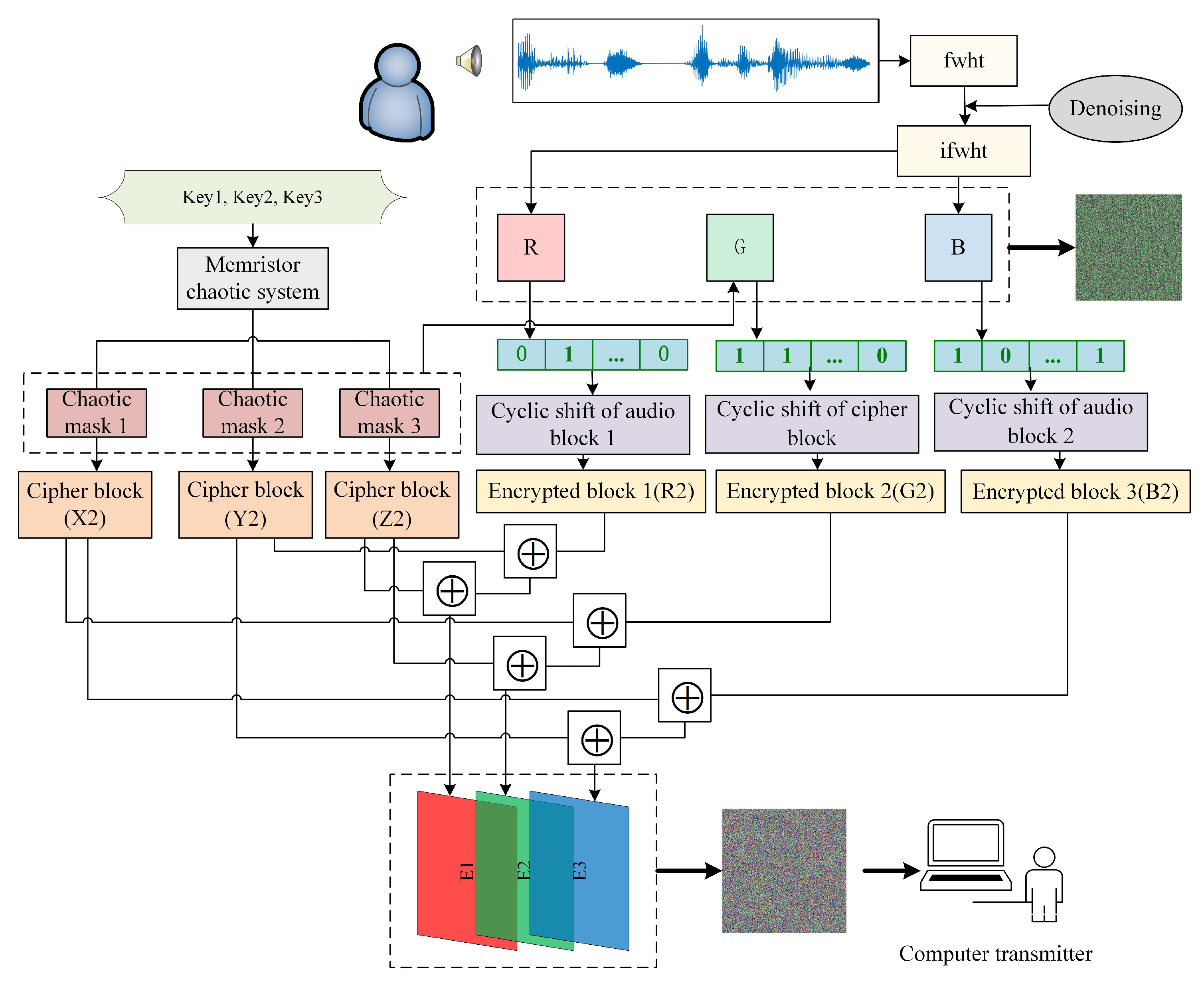Figure 7. Compression and encryption of audio signal: (a) original audio diagram; (b) compressed audio signal; (c) noise suppression; (d) reshaped audio signal; (e) reconstructed audio signal; (f) color audiogram; (g) color encrypted audio map; (h) decrypt audio map.
Figure 7. Compression and encryption of audio signal: (a) original audio diagram; (b) compressed audio signal; (c) noise suppression; (d) reshaped audio signal; (e) reconstructed audio signal; (f) color audiogram; (g) color encrypted audio map; (h) decrypt audio map.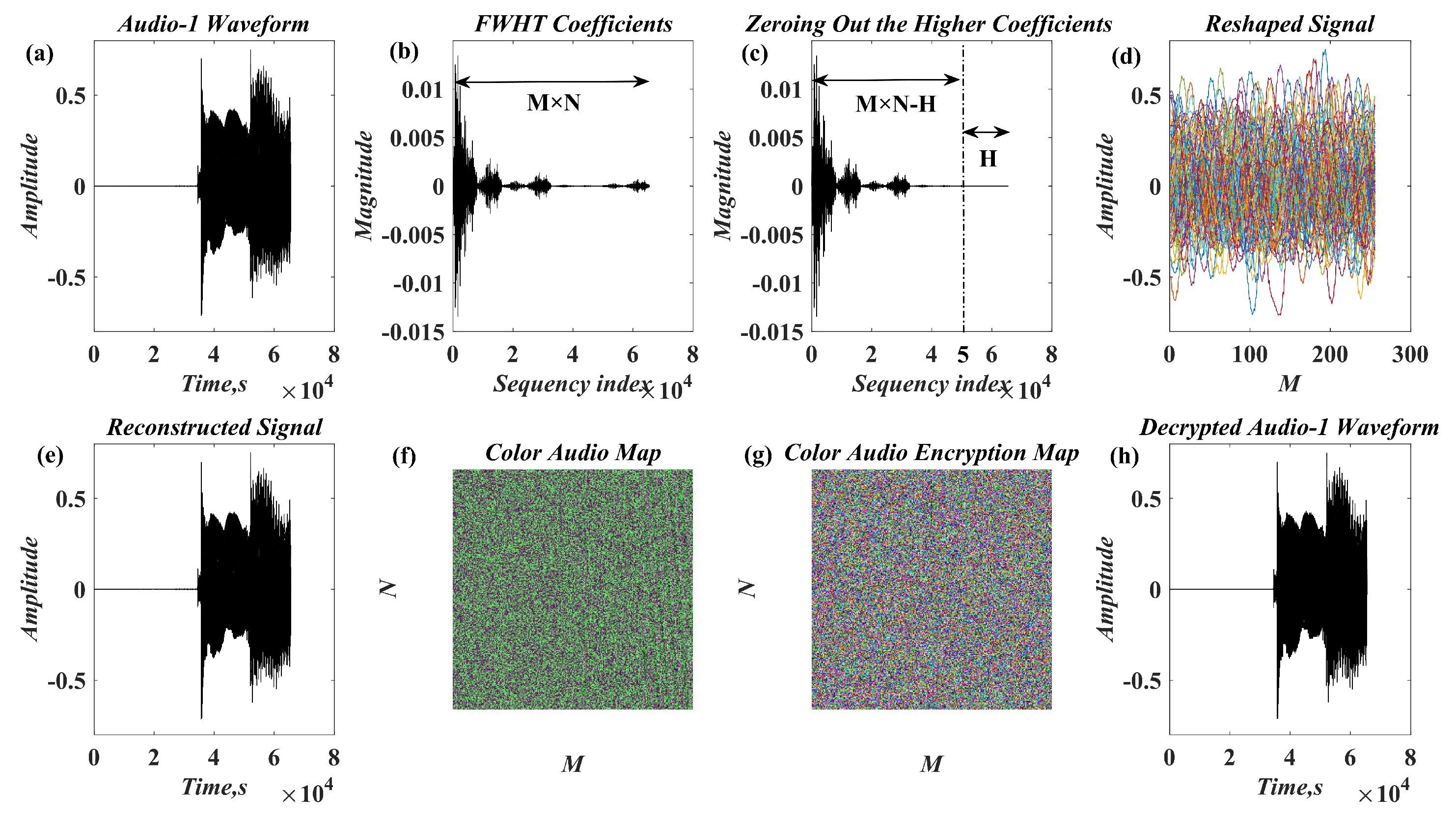Figure 8. Key sensitivity test: (a) Change Key 1; (b) change Key 2; (c) change Key 3; (d) Correct decryption.
Figure 8. Key sensitivity test: (a) Change Key 1; (b) change Key 2; (c) change Key 3; (d) Correct decryption.Figure 9. Histogram: (a) histogram of original audio signal; (b) histogram of encrypted audio signal.
Figure 9. Histogram: (a) histogram of original audio signal; (b) histogram of encrypted audio signal.Figure 10. Bar chart of RMS and CF values of original audio and encrypted audio. (a) RMS value (b) CF value.
Figure 10. Bar chart of RMS and CF values of original audio and encrypted audio. (a) RMS value (b) CF value.Table 1. Original information and encrypted information of different audio signals.
Table 1. Original information and encrypted information of different audio signals.
FilenameSize(MB)Original Audio WaveformEncrypted Graph
Audio 10.260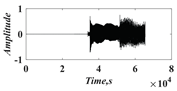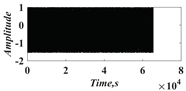Audio 20.128Audio 32.56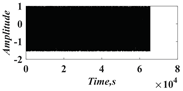Audio 41.05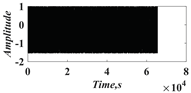Table 2. Autocorrelation of original audio, encrypted audio, and decrypted audio signals.
Table 2. Autocorrelation of original audio, encrypted audio, and decrypted audio signals.
FilenameOriginal Audio DataEncrypted Audio DataDecrypted Audio Data
Audio 1Audio 2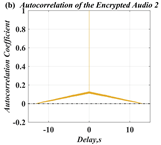Audio 3Audio 4Table 3. Distribution of adjacent samples of original audio and encrypted audio in horizontal and vertical directions.
Table 3. Distribution of adjacent samples of original audio and encrypted audio in horizontal and vertical directions.
FilenameOriginal AudioEncrypted Audio
HorizontalVerticalHorizontalVertical
Audio 1Audio 2Audio 3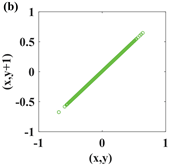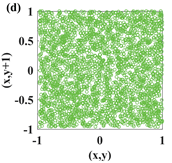Audio 4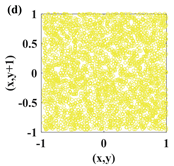Table 4. Correlation coefficient between raw audio and encrypted audio.
Table 4. Correlation coefficient between raw audio and encrypted audio.
FilenameOriginal AudioEncrypted AudioCorrelation Between Original
and Encrypted Audio
HorizontalVerticalHorizontalVertical
Audio 10.99251.0000−0.0012$4.5618 × 10 − 4$0.0014
Audio 20.96681.00000.0013$4.5302 × 10 − 4$0.0054
Audio 30.99211.0000$3.2348 × 10 − 4$−0.0026−0.0051
Audio 40.92171.00000.00430.00160.0021
Reference 0.9445−0.0081
Reference 0.99810.9981−0.00940.00790.0056
Table 5. Spectrum diagram.
Table 5. Spectrum diagram.
FilenameOriginal Audio DataEncrypted Audio DataDecrypted Audio Data
Audio 1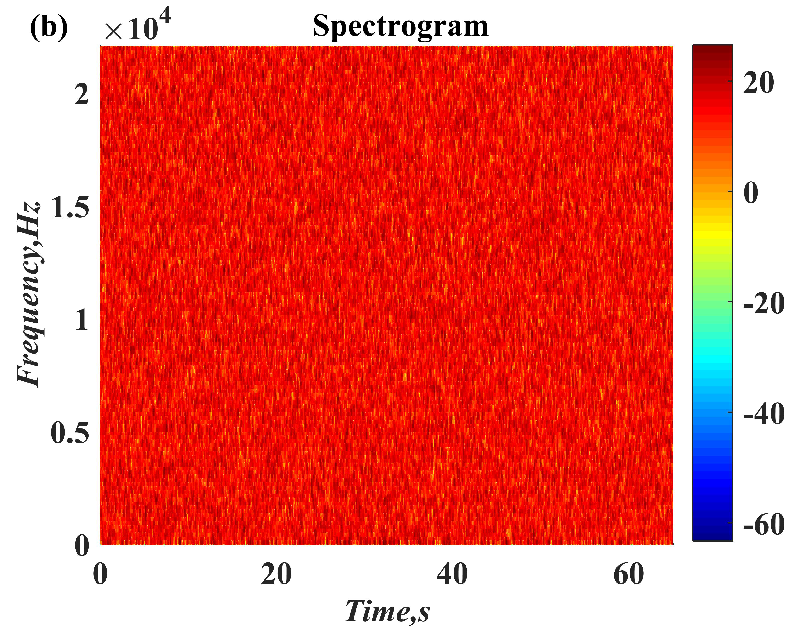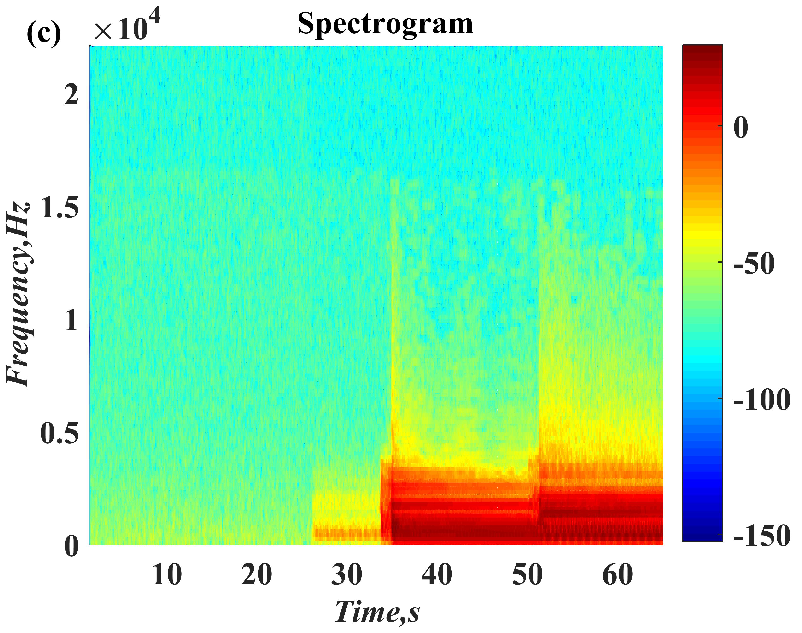Audio 2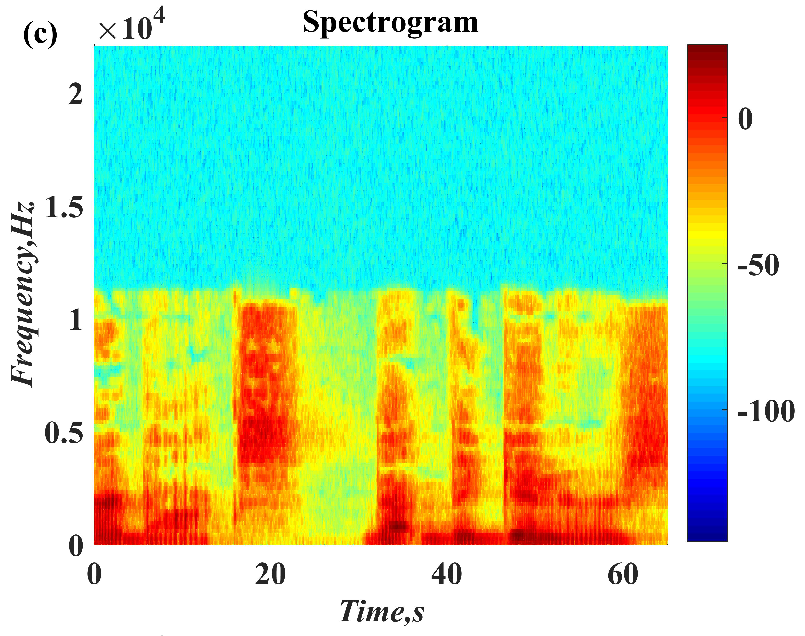Audio 3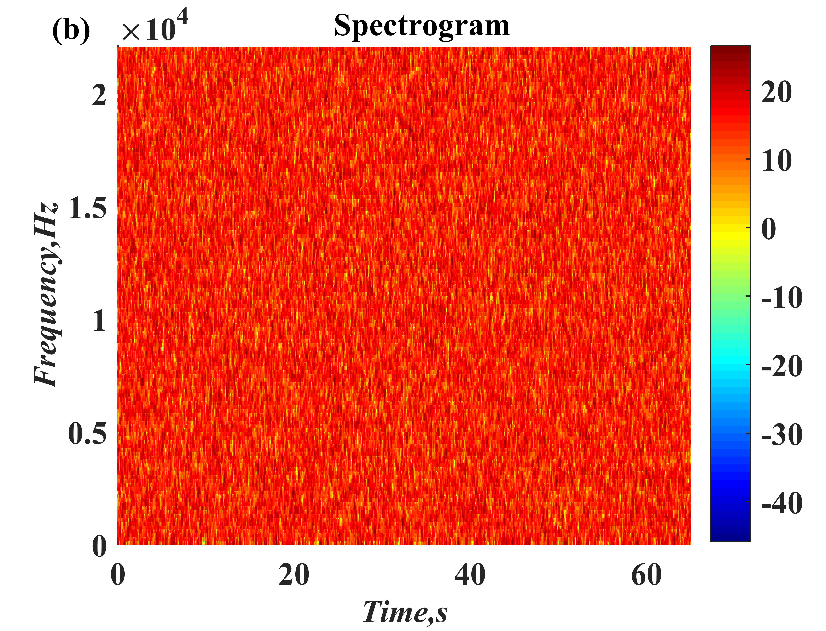Audio 4Table 6. NSCR and UACI values.
Table 6. NSCR and UACI values.
AudioNSCRUACI
Audio 199.9268%32.8195%
Audio 299.9695%32.7827%
Audio 399.8062%32.8285%
Audio 499.9786%32.8661%
Reference 99.9884%30.2437%
Reference 99.60812%36.39705%
Reference 99.996%
Table 7. Root Mean Square (RMS) and Crest Factor (CF).
Table 7. Root Mean Square (RMS) and Crest Factor (CF).
AudioSizeRMS (dB)CF (dB)Lossless Reconstruction
Audio 1260 KB0.138910.5234Yes
EAudio 1260 KB0.57013.4409
DAudio 1260 KB0.138910.5234
Audio 2128 KB0.053616.4115Yes
EAudio 2128 KB0.56863.4499
Audio 32.56 MB0.199811.0925Yes
EAudio 32.56 MB0.56753.4563
Audio 41.05 MB0.065215.5818Yes
EAudio 41.05 MB0.56933.4453
Reference 918 KB0.57864.7621Yes
Reference $≅ 0.6$No
Reference 1.84 MB0.60424.3754Yes
Table 8. SNR, PSNR and Encryption efficiency.
Table 8. SNR, PSNR and Encryption efficiency.
AudioSizeSNR (dB)PSNR (dB)Total (s)Speed (s/KB)
Audio 1260 KB−12.51834.63020.6364330.002448
Audio 2128 KB−20.55704.86590.3265670.002551
Audio 32.56 MB−9.56994.41865.3425000.002087
Audio 41.05 MB−18.88504.83462.5315560.002411
Reference 304 KB−28.14004.310058.630000.190000
Reference 439 KB−22.1394324.75001.1760000.002679
 Publisher’s Note: MDPI stays neutral with regard to jurisdictional claims in published maps and institutional affiliations.